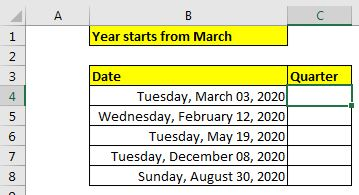# How to Determine Fiscal Quarter of Date in Excel

What is the Fiscal Quarter of Year?

A Fiscal Quarter of year is 1/3 part of the financial year of a company. Suppose a company yearly period is Feb'19-Jan'20, then the first fiscal quarter of the company's year will contain month's Feb'19, Mar'19, and Apr'19. Similarly, the second, third and fourth quarters will have the next 3 months of previous months.

You can also call it the financial quarter of a company.

## How to Calculate the Fiscal Quarter of Date in Excel?

To calculate the fiscal year of a given date in Excel we use this simple generic formula:

 =CHOOSE(MONTH(date),1,1,1,2,2,2,3,3,3,4,4,4)

Date: This is the date of which you want to get the fiscal quarter.

The numbers in the generic formula are strategically placed. The above generic assumes that the fiscal year start from Jan. If the fiscal year starts from Feb the number arrangement will be 4,1,1,1,2,2,2,3,3,3,4,4. Why? we will learn through examples.

### Example: Get the Fiscal Quarter/Financial Quarter of given dates when the fiscal year starts from March.

So, here we have some dates in excel range B4:B8. We are told that the year starts from the month of March.  So all dates in March, April, and May must come in the 1st quarter and so on.Using the above generic formula, we create the below excel formula to calculate the fiscal quarters of these dates.

 =CHOOSE(MONTH(B4),4,4,1,1,1,2,2,2,3,3,3,4)

For the first date, it returns 1 as 3rd March is surely in the first quarter of the financial year of the company that starts its year from March. And Feb is in the fourth quarter as it is the closing month of such an organization.

Souu...,  How does it work?

This is simple. The MONTH function returns the month of the suppled date. In the formula above, it returns 3 for the month march.

Next, the CHOOSE function returns the 3rd value from the array we have given. The 3rd value in the array 4,4,1,1,1,2,2,2,3,3,3,4 is 1.

Similarly, for the date of FEB month, the MONTH function returns 2. And the 2nd value in the array is 4.

So, yeah this is the mechanism of the formula for finding a fiscal quarter of the given date.

Yes! You are right. You can use the INDEX function to do this task. The formula will look like this.

 =INDEX({4,4,1,1,1,2,2,2,3,3,3,4}, MONTH(B4))

Here, we have used the same array in curly braces (you don't need to use CTRL+SHIFT+ENTER) to get the right quarter for the given date. The month function returns the number of the month of the given date. INDEX function uses that number as an index in the provided array and returns the quarter number.

So yeah guys, this how you can calculate fiscal quarter of the given date in Excel. I hope I was explanatory enough and it was helpful. If you have any doubt regarding this or any have any other Excel VBA related query, ask in the comments section below.

Relate Articles:

Terms and Conditions of use

The applications/code on this site are distributed as is and without warranties or liability. In no event shall the owner of the copyrights, or the authors of the applications/code be liable for any loss of profit, any problems or any damage resulting from the use or evaluation of the applications/code.